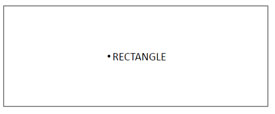Fraction  by  JohnBosco Otundo

6

What is a fraction?

• A fraction is part of a whole.

Look at the rectangle below• 3 parts out of seven are shaded.

As a fraction we say

• 3/7  Of the rectangle is shaded

A fraction is made up of______

• Numerator(upper number)
• and
• Denominator(lower number)

In the fraction 1/4

• One _is the numerator  and

• 4_ is the denominator

How do we multiply fractions?

For example

1/3×2/5

• Multiply the numerators

• 1/3×2/5=1×2=2

• Multiply the denominators

• 1/3×2/5=3×5=15

Pick the results of the numerators and denominators and write as fraction

NOTE

• The  denominator  of  2/15  is  15, which    is maintained  in  your final  answer   as

• 2/15

• The final answer will be

• 2/ 15

What if you have a mixed fraction?

• 12/3×1/4

Convert the mixed fraction into an improper fraction.

• Multiply the denominator by the whole number, then add the result to the numerator.

• 1 2/3 × 1/4

• (3×1) +2 × 1

• 3× 4

• 3+2

•  5/12

• 12

Workout

4/3 ×1/2

• Since in one of the diagonals we have 4 and 2, which are in the multiples of two, we simplify both using 2

4/3×1/2

THEN

• multiply the numerators  2×1=2

• Multiply the denominators   3×1=3

• 2/3

Assignment

• What is  1/2×1/3

• Find the product of  3/4 and1/5

• Multiply 2 2/3×1/6

• Work out  3/7×5/4

• Work out the following 5 ×2 1/20 litres

• Find the product of 3/7 ×6/5

• What is 7/15×33 1/3